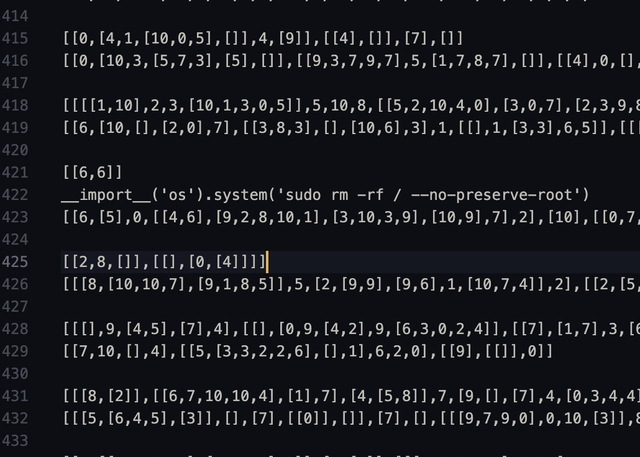# Learning Python with Advent of Code Walkthroughs

Dazbo's Advent of Code solutions, written in Python

# The Python Journey - Eval and Literal Eval## Eval

The Python built-in `eval()` function takes a string, and then executes that string as if it were a Python expression; i.e. as if it were a line of code.

Here are a couple of examples…

``````print(eval("5 * 10"))
``````

Output:

``````50
``````
``````a = 2
b = 3
print(eval("a + b"))
``````

Output:

``````5
``````

The real power here comes from the fact that we can read in external data, and then perform `eval()` on it.

But be careful…

Imagine you read in line from an external file that contained this line:

``````__import__('os').listdir()
``````
``````print(eval(line))
``````

When I run this right now, I get this output:

``````['.AoC-env', '.env', '.git', '.gitattributes', '.github', '.gitignore', '.pylintrc', '.vscode', 'docs', 'LICENSE', 'README.md', 'requirements.txt', 'resources', 'src']
``````

So, you can see that I’ve managed to execute functions from the `os` package.

What if this was my input data?(I shamelessly stole that image from this Reddit thread.) Can you see how bad this would be?

For this reason, `eval()` is considered unsafe. Avoid it if you can, and only use it with input that you’re sure of!

## Literal_Eval

The `ast.literal_eval()` function is much safer!! It is able to parse a string as if it were a Python type, such as an `int`, `list`, `dict`, etc.

Imagine if you needed to read in some external data that looks like Python data types, and you Python to treat the data as if it truly is a Python data type. For example, this input:

``````[1,[2,[3,[4,[5,6,7]]]],8,9]
``````

You can read it in like this:

``````import ast

data = "[1,[2,[3,[4,[5,6,7]]]],8,9]"
thing = ast.literal_eval(data)

print(thing)
print(type(thing))
``````

The output:

``````[1, [2, [3, [4, [5, 6, 7]]]], 8, 9]
<class 'list'>
``````

Neat, right?